Next: Transformation of Lagrange brackets Up: Derivation of Lagrange planetary Previous: Preliminary analysis

# Lagrange brackets

Six new equations can be derived from Equations (G.24)-(G.29) by multiplying them successively by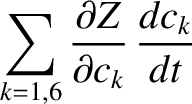,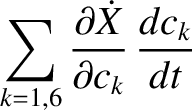,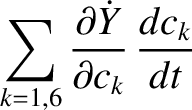,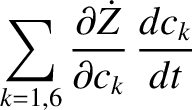,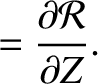, and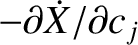, and then summing the resulting equations. The right-hand sides of the new equations are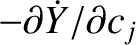(G.30)

The new equations can be written in a more compact form via the introduction of Lagrange brackets, which are defined as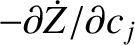(G.31)

where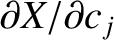,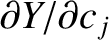, and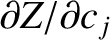. Thus, the new equations become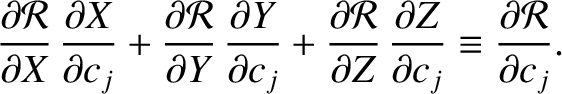(G.32)

for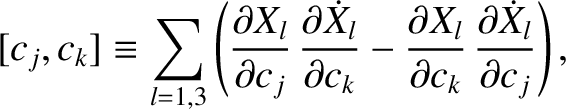. Note, incidentally, that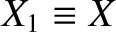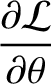(G.33) and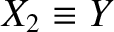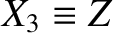(G.34)

Let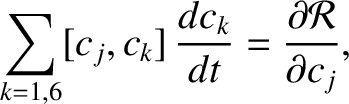(G.35)

where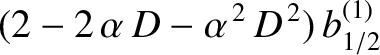and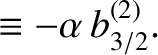are any two orbital elements. It follows that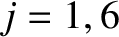(G.36)

or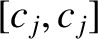(G.37)

However, in the preceding expression,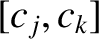and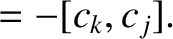stand for coordinates and velocities of Keplerian orbits calculated with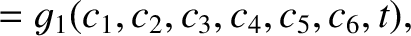treated as constants. Thus, we can write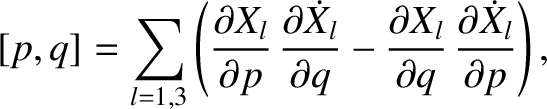and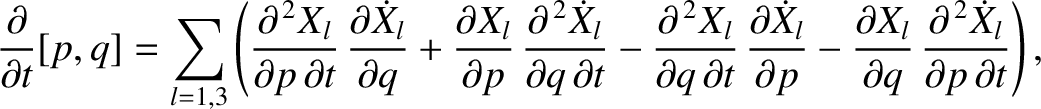, giving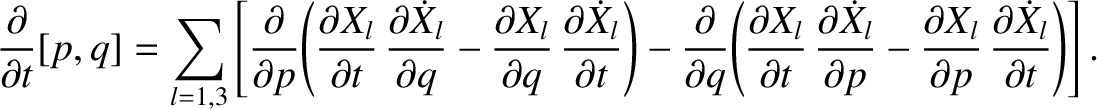(G.38)

because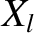(G.39)

where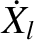. Expression (G.38) reduces to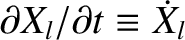(G.40)

where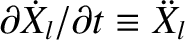. Hence, we conclude that Lagrange brackets are functions of the osculating orbital elements,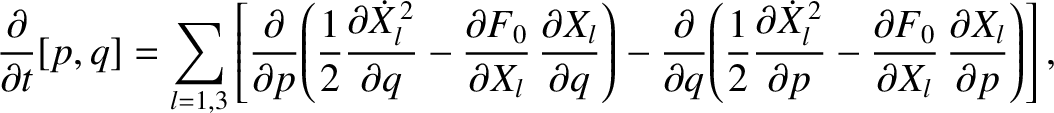, but are not explicit functions of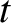. It follows that we can evaluate these brackets at any convenient point in the orbit.Next: Transformation of Lagrange brackets Up: Derivation of Lagrange planetary Previous: Preliminary analysis
Richard Fitzpatrick 2016-03-31